# How does a stepper motor work?

Hi friends,

The stepper motors convert electrical pulses received by their excitation (control) winding into discrete angular displacements-commonly called as steps.

The characteristic property of the stepper motor is the step-by-step turning of the motor shaft. One complete turn of the shaft is made up from an exactly specified number of steps, which is determined by the motor design. This property meets the requirement for operating directly from digital signals. The stepper motor can thus be the bridge between digital information and incremental mechanical displacement.

Since there is a direct correspondence between the number of input pulses and the number of discrete angular steps taken by the motor, there is no need for a feedback sensor for measuring the angular position of the rotor. This means that automatic control system can be built without feedback using a stepper motor as actuators.

Stepper motors are widely are widely used in numerically controlled (NC) machine tool positioning systems, since

• The command signal in NC systems is in the form of digital pulses and a stepper motor can operate directly from such command signals.
• There is no need for feedback sensor which is quite difficult cumbersome to install on machine tools.
• The open loop system does not pose stability problems, thereby, considerably simplifying the system design.

The stepper motors are often constructed with a multipole, multiphase stator winding similar to the conventional electrical machines. They usually have three or four phase windings wound for a number of poles determined by the desired angular displacement per input pulse.

The rotor is either of permanent magnet type or the variable reluctance type. The absence of rotor winding, commutator and carbon brushes, makes stepper motor quite robust and reliable.

# How does a stepper motor work?

Stepper motors are actuated by means of an external drive logic circuit. As a series of pulses of voltage is applied to the input of the drive circuit, it feeds suitable currents to the stator windings of the motor to make the axis of the magnetic field step around in synchronism with the input pulses. And this causes rotation in the rotor in synchronism with the input pulses.

## Step Angle

It is defined as the angular displacement of the rotor in response to each pulse.

Step angle = Difference of the rotor pitch and the stator pitch

Where, Stator pitch = 360/Number of stator poles
Rotor pitch = 360/Number of rotor poles

Smaller the step angle, greater the number of steps per revolution and higher the resolution or accuracy of positioning obtained.

The step angles can be as small as 0.72o or as large as 90o. But the most common step sizes are 1.8o, 2.5o, 7.5o and 15o.

## Types of Stepper Motor

There are several types of stepper motor currently available and can be classified into following three basic categories:

• Variable Reluctance Stepper Motor
• Permanent Magnet Stepper Motor
• Hybrid Stepper Motor

## Variable Reluctance Stepper Motor Working

Its rotor is made out of slotted steel laminations and has no winding in it. The stator usually is wound for three phases.

The stator windings are excited with the help of an external circuit in a specified sequence and the rotor seeks that position in which the reluctance between the stator and the rotor is minimum.

Figure shows a schematic representation of a variable reluctance stepper motor having six salient poles (teeth) with exciting winding around each of them. The rotor has four salient projections only.

A circuit arrangement for supplying current to the stator coils in proper sequence is shown in Figure.

The coils wound around diametrically opposite poles are connected in series such that when one tooth becomes an N-pole the other one becomes an S-pole.

Thus the three circuits (phases) are formed and they are energized from a DC source through an electronic switching device. For the simplicity, switches are shown as mechanical switches in Figure.

Now in this motor,

Stator pitch = 360/6 = 60o
Rotor pitch = 360/4 = 90o

Therefore, Step angle, β = 90 – 60 = 30o

The step angle of this motor is 30o. It means it will move 30o on every application of stator pulse and will take 360/30 = 12 steps to make a complete revolution.

## 1-phase ON or Full-step Operation

In this mode of operation, one stator phase is excited at one time.

When coil A – A’ is energized by closing switch S1, the rotor is subjected to an electromagnetic torque and rotates until its axis coincides with the axis of MMF set up by phase A and takes the position indicated in Figure (a).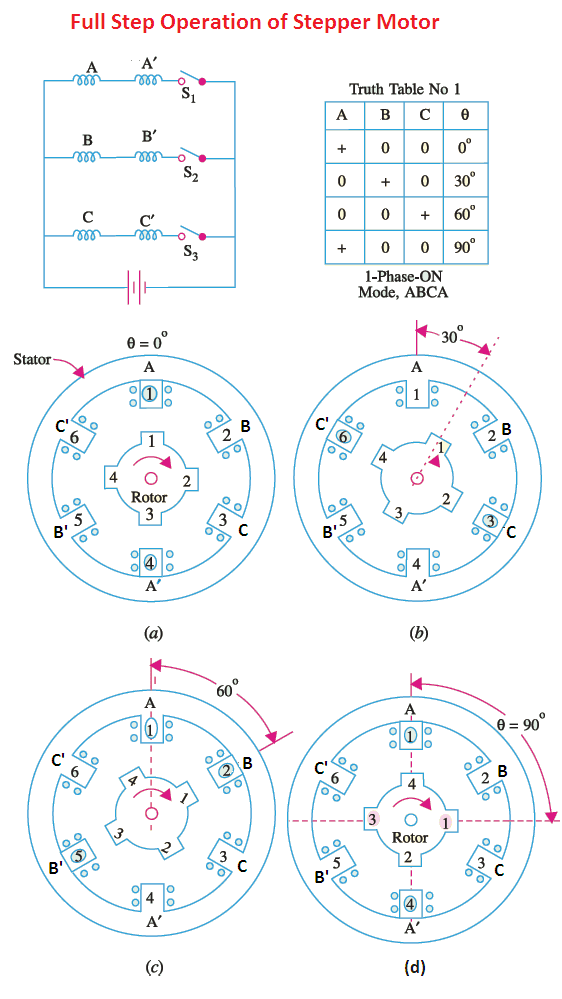When coil B – B’ is energized by closing switch S2 and opening S1, the rotor moves through a full-step of 30o (step-angle) in the clockwise direction and takes the position indicated in Figure (b).

Similarly, when coil C – C’ is energized by closing switch S3 and opening S2, the rotor moves through a further step of 30o in the clockwise direction and takes the position indicated in Figure (c).

Next, when coil A – A’ is energized again by closing switch S1 and opening S3, the rotor rotates through a further step of 30o in clockwise direction and takes the position indicated in Figure (d).

By now the total angle turned is 90o. As each switch is closed and the preceding one opened, the rotor each time moves through a step of 30o.

By successively closing the switches in the sequence 1-2-3-1 and thus exciting stator phases in sequence ABCA etc. the rotor will rotate clockwise in 30o steps.

If the switching sequence is reversed i.e. 3-2-1-3, the rotor will rotate in the anticlockwise direction in 30o steps.

## 2-Phase-ON Mode

In this mode, two stator phases are energized at a time.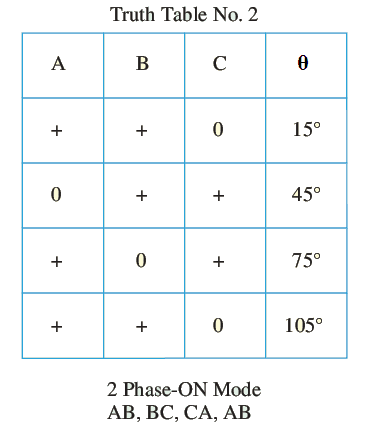When the two phases are excited simultaneously, the rotor is subjected to an electromagnetic torque from both phases and comes to rest at a point midway between the two adjacent full-step positions.

If the stator phases are energized in the sequence of AB, BC, CA etc. , the motor will move in full steps of 30o (as in the 1-phase mode) but its rest positions will be at the midpoint of the full-step positions.

## Half–step Operation

If we energize the stator in the sequence A, AB, B, BC, C etc. i.e. alternately in the 1-phase-ON and 2-phase-ON modes the rotor will move in half step angles (30/2 = 15o in this case) each time.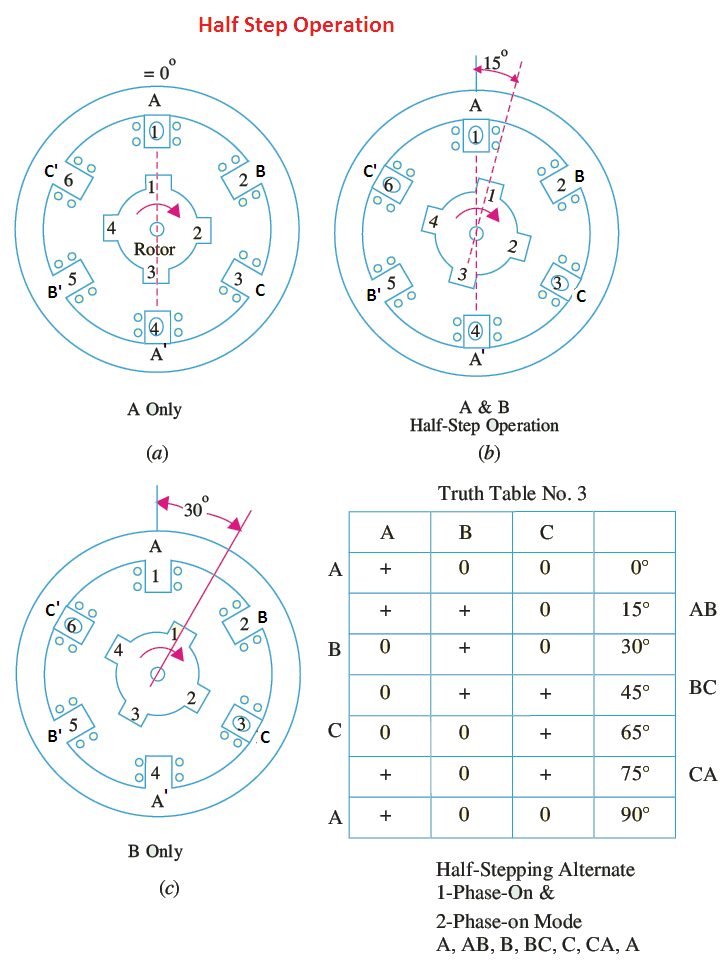# Permanent Magnet Stepper Motor Working

The rotor made out of permanent magnet material is either of a salient pole or cylindrical type. The stator has a two or three or four phase winding located in a slotted structure. The number of slots per pole per phase is usually chosen as one in multipolar machines. Permanent magnet stepper motors have found the widest application because they have good dynamic and static characteristics and a relatively high efficiency.

Figure shows a schematic representation of a 2-pole, 2-phase permanent magnet stepper motor. In this case,

Rotor pitch = 360/2 = 180o

Stator pitch = 360/4 = 90o

Therefore, step angle β = 180 – 90 = 90o

Since in this case, step angle is 90o, therefore this motor is capable of making discrete steps of 90o as soon as voltage pulses are applied to the two phases of the exciting winding in a specified sequence.

The axis of the magnetic field can have four different positions corresponding to two different directions of flow of current in phases A and B of the exciting winding.

As a result of interaction between the magnetic fields caused by the exciting winding and the permanent magnet, electromagnetic torque is produced in such a way as to make the rotor follow the axis of the stator magnetic field.

Hence, the application of each voltage pulse to the exciting winding makes the axis of the stator field shift by 90o at every switching, thus causing the rotor to make discrete angular displacements of 90o.

If the direction of current flow in any one of the phases of the exciting winding is reversed, keeping the sequence of switching same, the direction of rotor movement would be reversed.

## 1-Phase ON Mode

In this mode of operation, only one phase is energized at a time.
Consider the figure shown above, here, phase A is energized with positive current ia+. Here, θ = 0o, rotor moves to the position shown in Figure (a).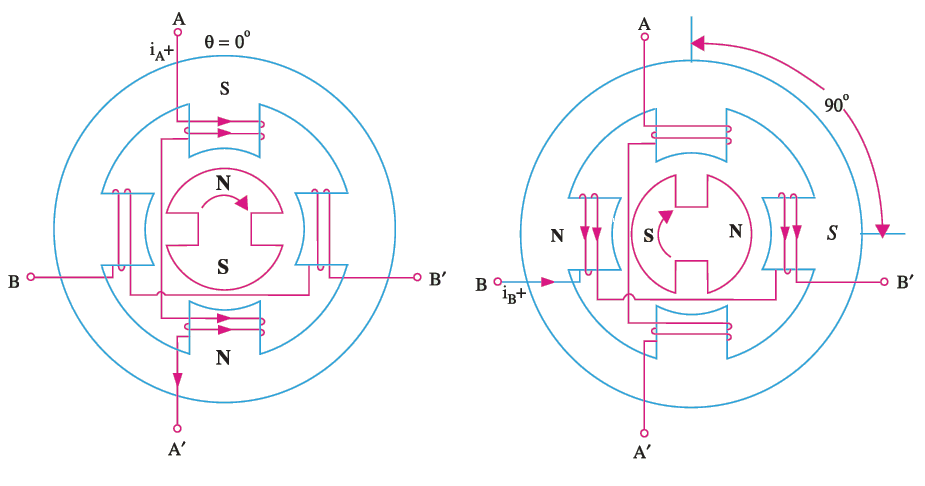Thereafter, phase B is energized with positive current ib+, the rotor moves a full step of 90o in the clockwise direction.

Next, phase A is energized again but with negative current ia, the rotor takes another a full step of 90o in the clockwise direction.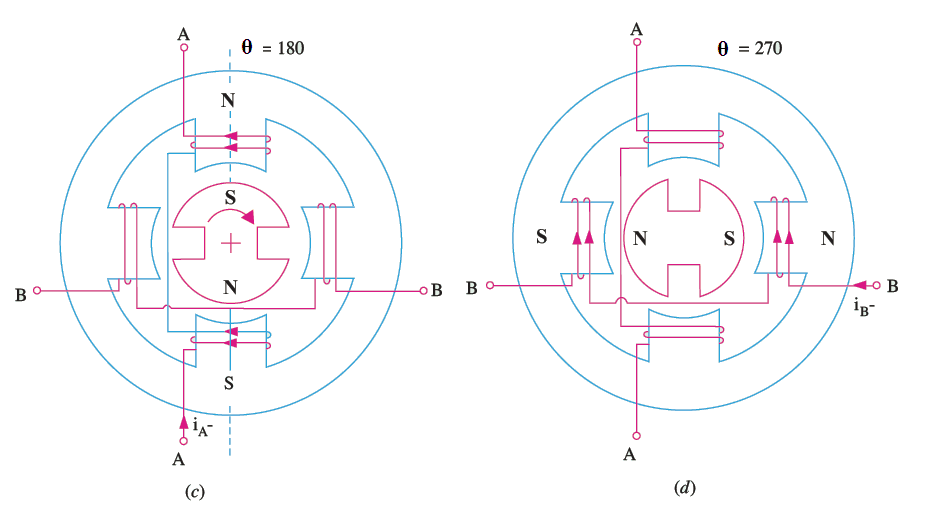Similarly, phase B is energized again but with negative current ib, the rotor takes another a full step of 90o in the clockwise direction.

After this, phase A is energized with positive current ia+, the rotor rotates further a full step of 90o in the clockwise direction.

In this way, the rotor completes one revolution of 360o.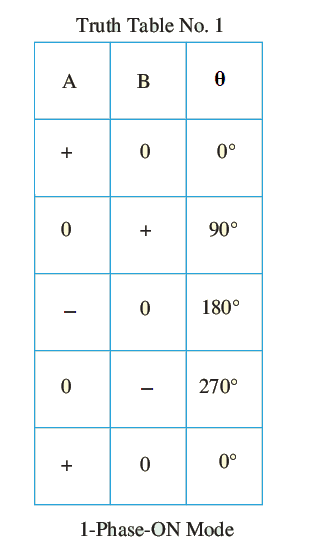## 2-Phase ON Mode

In this mode of operation, both the phases are energized simultaneously. In this mode, resulting steps are of the same size (i.e. 90o) but the rotor pole rests between the two adjacent full-step positions.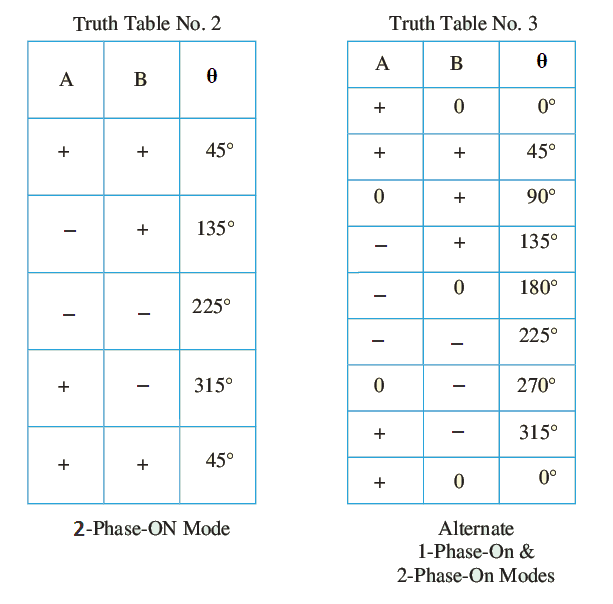## Half Step Operation

In this mode, 1-phase ON and 2-phase ON modes are used alternatively. The step size becomes half of the full step (45o in this case) thereby increasing the resolution.

Also read: Stepper Motor Working Principle

© www.yourelectricalguide.com/ How does a stepper motor work?

### 2 thoughts on “How does a stepper motor work?”

1.thanks for post,I want know about proximity pnp npn sensor

1.Ajay Sharma

Thank you Salam for your feedback. I have to consider many points before publishing a post. Therefore it is not possible to publish a post on request. So I am unable to help more.